## How to Split Date into Day, Month and Year in Excel

If you have a date column in your Excel spreadsheet and need to separate it into day, month, and year, you may be wondering how to do so efficiently. This post will guide you through four different methods for splitting a date into day, month and year using Excel formulas, Text to Columns, Flash Fill, and VBA Code. How do I quickly split date as Day, Month and Year using Formulas or Text to Columns feature in Excel.

## 1. Split Date into Day Month and Year with Formulas

Assuming that you have a list of data in range A1:A6, which contain date values. And you want to split each date value into three separate columns for day, month and year. How to achieve it. You can use three formulas based on the DAY function, the Month function and the Year function to achieve the result of splitting dates. Just do the following steps:

Step1: type Day string in a blank cell in a new column, such as: B1, and type Month string in Cell C1, type Year string in Cell D1.

Step2: Type the following formula in Cell B2, and press Enter key, then drag the AutoFill Handle over to other cells to apply this formula.

``=DAY(A2)``

This formula will extract Day Value from the given date in range A2:A6.

Step3: Type the following formula based on the Month function to get Month value from date in Cell C2, and press Enter key, and then drag the AutoFill Handle over to other cells to apply this formula.

``=MONTH(A2)``

This formula will extract Month value from the given date in range A2:A6

Step4: Type the following formula in Cell D3 to get the Year value, and press Enter key, and then drag the AutoFill Handle over other cells.

``=Year(A2)``

You should see that all dates in range A2:A6 have been split into separate day, month and year.

## 2. Split Date into Day Month and Year with Text to Column Feature

You can also use Text to Column options to achieve the same result of splitting date into separate day, month and year in three columns. You just need to do the following steps:

Step1: select the range of cells that you want to split.

Step2: go to DATA tab, click Text to Columns command under Sort & Filter group. And the Convert Text to Columns Wizard dialog will open.

Step3: select Delimited as the file type, and click Next button.

Step4: only check Other checkbox under Delimiter section, type the delimiter / into the Other text box, and click Next button.

Step5: select one Destination cell, such as: B2, click Finish button.

Step6: the date column has been split into separate day, month and year.

## 3. Split Date into Day, Month and Year with Flash Fill Feature

Flash Fill can quickly and easily split a date column into day, month, and year components in Excel. Just do the following steps:

Step1: First select three new columns to the right of the date column.

Step2: In the first new column, enter the first value you want to extract. For example, the day value.

Step3: In the second new column, enter the second value you want to extract. For example, the month value.

Step4: In the third new column, enter the third value you want to extract. For example, the year value.

Step5: Click on the first day value in the first new column, then click on Flash Fill command under Data Tools group in Data tab.

Step6: Excel will automatically show the suggested day values in the remaining cells of the new column.

Step7: Repeat step 6 for the remaining two new columns to extract the month and year values.

Once you have extracted all three values, you can delete the original date column and the temporary columns containing the extracted values.

## 4. Split Date into Day, Month and Year with VBA Code

You can also use VBA code to split a date into day, month, and year components in Excel. Just do the following steps:

Step1: Open your Excel workbook and press ALT + F11 to open the Visual Basic Editor.

Step2: Click on “Insert” from the top menu and choose “Module” to create a new module.

Step3: Copy and paste the following code into the module, Save the module and close the Visual Basic Editor.

```Sub SplitDate_excelgeek()
Dim dateRange As Range
Set dateRange = Application.InputBox("Select the range of cells containing the dates to split", Type:=8)
Dim cell As Range
For Each cell In dateRange
Dim dayValue As Integer
Dim monthValue As Integer
Dim yearValue As Integer
dayValue = Day(cell.Value)
monthValue = Month(cell.Value)
yearValue = Year(cell.Value)
cell.Offset(0, 1).Value = dayValue
cell.Offset(0, 2).Value = monthValue
cell.Offset(0, 3).Value = yearValue
Next cell
End Sub```

Step4: Click on “Macros” under the “Code” group. Select the “SplitDate_excelgeek” macro from the list and click on “Run“.

Step5: select the range of cells containing the date values that you want to split. For example, select range of cells A2:A5.

Step6: The VBA Macro will split the selected date column into day, month, and year components and place the results in the adjacent columns.

## 5. Video: Split Date into Day Month and Year

This video tutorial will guide you on how to split a date into day, month, and year components using various methods in Excel.

## 6. Conclusion

Whether you need to work with a large dataset or just a few dates, these methods can help you quickly and accurately separate your date values into separate columns.

## 7. Related Functions

• Excel YEAR function
The Excel YEAR function returns a four-digit year from a given date value, the year is returned as an integer ranging from 1900 to 9999. The syntax of the YEAR function is as below:=YEAR (serial_number)…
• Excel MONTH function
The Excel MONTH function returns the month of a date represented by a serial number. And the month is an integer number from 1 to 12. The syntax of the MONTH function is as below:=MONTH (serial_number)…
• Excel DAY function
The Excel DAY function returns a day of a date (from 1 to 31).The DAY function is a build-in function in Microsoft Excel and it is categorized as a DATE and TIME Function.The syntax of the DAY function is as below:= DAY (date_value)…

## Add Months To Date In Excel

It is important to adjust time periods when performing financial modeling. In Excel, you can use the MONTH function to add a specific number of months to a date. The MONTH function takes one argument: the number of months you want to add. Here will show you how to add months to date in excel and even provide some examples that might be useful!

## Method1: Add Months to Date using EDATE Function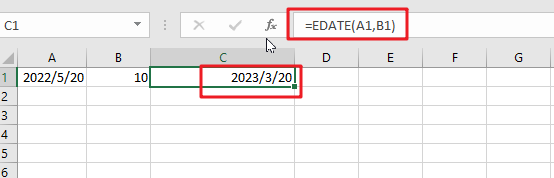The generic formula is:

`=EDATE(Sart_date, months)`
• `Start_date`: the starting date on which you want to add months
• `Months`: the month that you wish to add

Return Value:

The function will return the date that is the indicated number of months after the `start_date`. If you enter a negative number of months, it’ll count back from the end date.

When you enter the MONTH function, Excel looks at the date in the cell that you specify as the `start_date` argument. It then adds the number of months that you entered as the second argument to that date. If you enter a negative number for the second argument, Excel subtracts that number of months from the `start_date`.

To add a number of months is placed at B2 and add months to the date in cell A2. The formula for this would be:

`=EDATE(A1,B1)`Or simply enter the number you want in your formula, and it will be replaced with that value.

`=EDATE(A1,10)`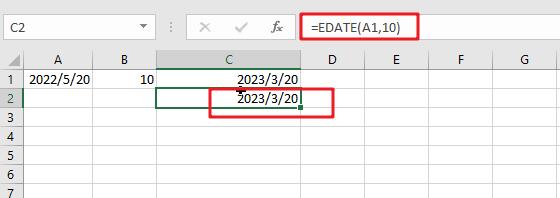### Explanation

This will add 10 months to the date in cell A1. The function will return the date that is the indicated number of months after the start_date. If you enter a negative number of months, it’ll count back from the end date.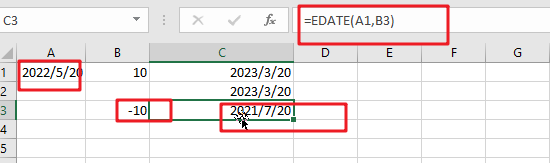You can also format the date in a certain way you want by right-clicking on it and then choosing ‘Format Cells’. In this pipping format, dialog select one date format type you want to display results. Then, press the Apply button to save changes made after filling out fields with proper data values following desired specifications, including today’s date if necessary!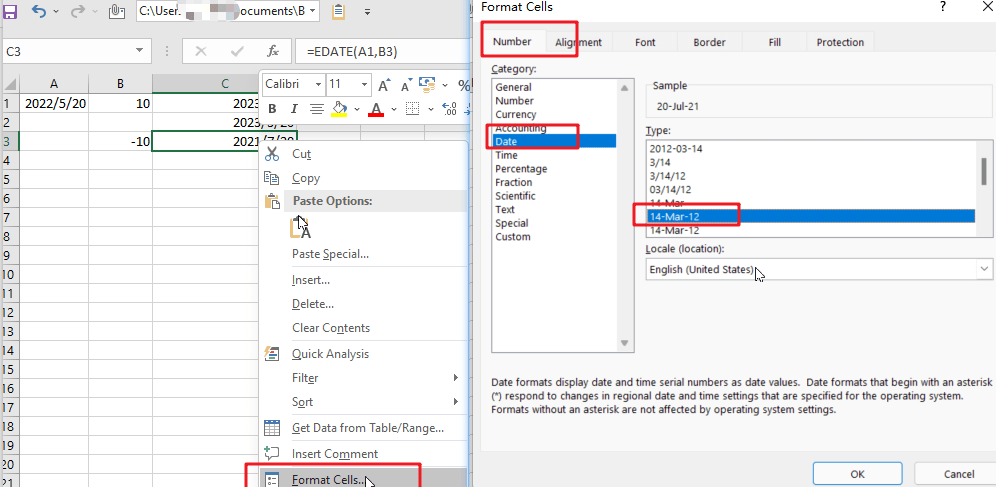The EDATE function is a great way to find out your next date. Simply enter any valid date and then select how many months away from now you want the new one! It’s really easy; just feed it with an amount less than or equal to the number of desired days between these two points-and voila.

Note:

Excel stores data as sequential serial numbers, which can be used in calculations. For instance, if you want to find out what day of the week it was on January 1st, 1900, then simply enter “serial#” into your formula bar and press Enter! The result will show that this date falls exactly 120 years ago – having been achieved by entering 44219 days after 1900 (which would make sense considering we’re talking about a Gregorian calendar).

## Method2: Add Months to Date using Date Function

The generic formula is:

`=DATE(YEAR(date),MONTH(date))+months,DAY(date)))`

### Arguments

• `Date`: The starting date on which you want to add months.
• Months: Months are important when adding or subtracting a certain number of months from/to dates. They add the indicated amount, depending on whether it’s positive and negative values respectively; for example, -1 means one month less than what you want (in other words: ago).
• YEAR(date): The year component of the date in Date.
• MONTH(date): The month component of the date in Date.
• `DAY(date)`: The day component of the date in Date.

### Return Value:

A date is the indicated number of months after the start_date. If you enter a negative number of months, it’ll count back from the end date.

### How This Formula Work

To add months to the date in cell A1, and a number of added is placed at B1. The formula for this would be as follows:

`=DATE(YEAR(A1),MONTH(A1)+B1,DAY(A1))`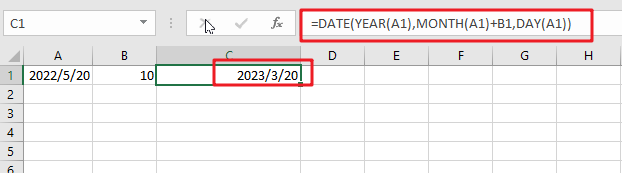With the Year, Month, and Day functions, you can easily create your date from any combination of these three values. With the Date function, you can create dates based on any year and month of your choice.

### Related Functions

• Excel DAY function
The Excel DAY function returns a day of a date (from 1 to 31).The DAY function is a build-in function in Microsoft Excel and it is categorized as a DATE and TIME Function.The syntax of the DAY function is as below:= DAY (date_value)…
• Excel EDATE function
TThe Excel EDATE function returns the serial number that represents the date that is a specified number of months before or after a specified date.The syntax of the EDATE function is as below:=EDATE (start_date, months)…
• Excel DATE function
The Excel DATE function returns the serial number for a date.The syntax of the DATE function is as below:= DATE (year, month, day)…
• Excel MONTH Function
The Excel MONTH function returns the month of a date represented by a serial number. And the month is an integer number from 1 to 12.The syntax of the MONTH function is as below:=MONTH (serial_number)…
• Excel YEAR function
The Excel YEAR function returns a four-digit year from a given date value, the year is returned as an integer ranging from 1900 to 9999. The syntax of the YEAR function is as below:=YEAR (serial_number)…

## Add Days to Date in MS Excel

If you are an vavid MS Excel user, then you might have come across situations where you need to add the same or different days into the particular date, and if you have done this task manually, then let me add that it’s the most impractical way to do it, especially when MS Excel has the functionality to do it in just a matter of seconds.

So by reading this article carefully, you would get to know different ways to add days into the Date in MS Excel in a few seconds.

So let’s dive into it,

## Ways To Add Days To Date

There are three most excessively used ways to add days to date in MS Excel, stated as follows:

1.    Adding Days to Dates by Value

2.    Adding Days from a specific Cell Location

3.    Adding Days using the DATE() Function

## 1. Adding Days to Dates by Value

Assume that you want to add a predetermined number of days (say, 10) to each date in column A and save the result in column F as shown below: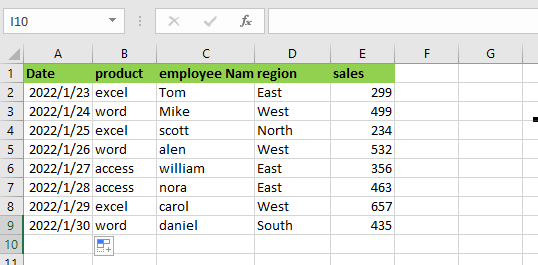So for this, you need to do the steps are given below:

Step1: Please select the first cell of the column where you want to save the result (in our example, cell F2).

Step2: Enter ‘`=`‘ and then choose the first cell in the column holding the dates to which you want to add days (cell A2).

Step3: Then, enter ‘`+`‘ followed by the number of days you wish to add. So, to add 10 days, enter ‘`+10`‘ in the same box. This suggests that the formula for cell F2 should be `=A2+10`.

Step4: On your keyboard, press the Enter In cell F2, you will obtain the result of adding 10 days to the date.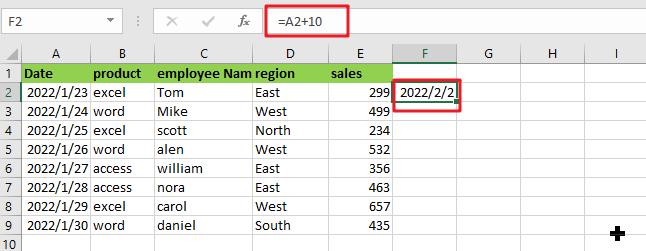Step5: If you want to add the same number of days to all of the dates in Column A, then just pull down the fill handle (at the bottom right of cell F2) to replicate the formula to all of the rows in Column F.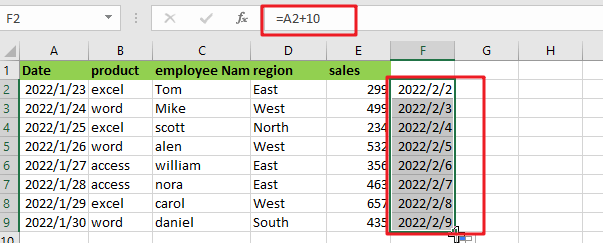## 2.  Adding Days From A Specific Cell Location

If you want to add a different number of days to each date, then put the number of days (which is needed to add) in a separate column for each row (as shown below).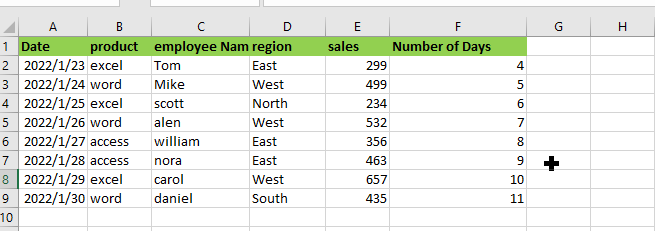Then, follow the instructions outlined below:

Step1: Please select the first cell of the column where you want to save the result (in our example, cell G2).

Step2: Enter `=`‘ and then choose the first cell in the column holding the dates to which you wish to add days (cell A2).

Step3: After that, type `+`‘.

Step4: Then, in our example, choose the first cell in the column showing the number of days to add (cell F2). This suggests that the formula in cell G2 should be `=A2+F2`.

Step5: On your keyboard, press the Enter Add the number of days in cell F2 to the date in cell A2 to get the result.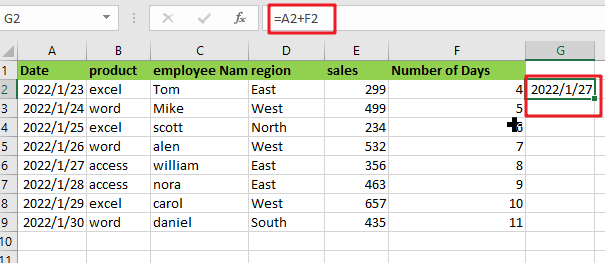Step6: Drag down the fill handle (located at the bottom right of cell G2) to duplicate the formula to all Column G’s rows. This will add the cell in column F to the cell in column A for each row.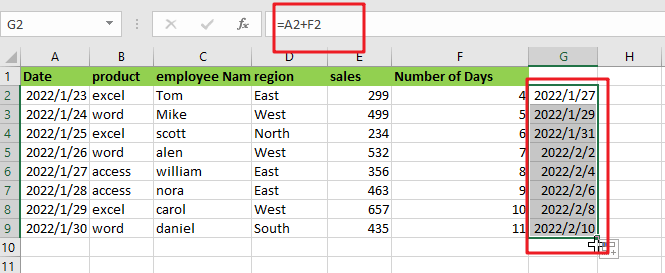Note: Your result may show as a serial number rather than a date in rare situations. This may occur if the format of your result cell is Number or Text. In such circumstances, you may easily convert the serial to a Date format using the following formula:

• Select the cell or the entire column using the right mouse button.
• From the popup menu, choose Format Cells. The Format Cells dialogue box will appear.
• On the Number tab, choose the date from the Category drop-down menu.
• From the Type list on the right side of the dialogue box, choose the format in which you want your date to display.
• Select OK.

This would change the format of all your cells to date.

## 3. Using the DATE() Function to Add Days

There is another method for adding days to a date in Excel. This is accomplished by using the DATE function. Let’s utilize the same dataset as we used before:What you must do is as follows:

Step1: Please select the first cell of the column where you want to save the result (in our example, cell F2).

Step2: Fill up the blanks using the following formula: =DATE(YEAR(A2), MONTH(A2), DAY(A2)+10)

Step3: On your keyboard, press the Enter In cell A2, you will obtain the result of adding 10 days to the date.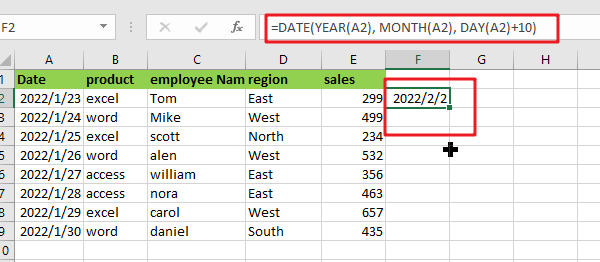Step4: Drag down the fill handle (located at the bottom right of cell F2) to replicate the formula to all of Column F’s rows.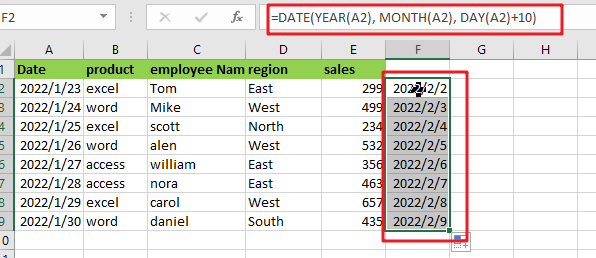You may even add months and years to your date using this approach. Assume you wish to add one year, three months, and four  days to a date. You may simply achieve this using the approach described above.

Simply add 1 to the first parameter, 3 to the second, and 4 to the third, and your formula becomes:

`=DATE(YEAR(A2)+1, MONTH(A2)+3, DAY(A2)+4)`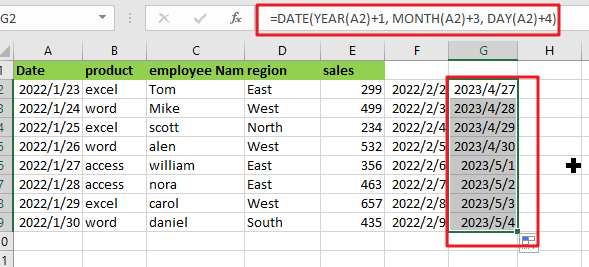### Related Functions

• Excel DAY function
The Excel DAY function returns a day of a date (from 1 to 31).The DAY function is a build-in function in Microsoft Excel and it is categorized as a DATE and TIME Function.The syntax of the DAY function is as below:= DAY (date_value)…
• Excel DATE function
The Excel DATE function returns the serial number for a date.The syntax of the DATE function is as below:= DATE (year, month, day)…
• Excel MONTH Function
The Excel MONTH function returns the month of a date represented by a serial number. And the month is an integer number from 1 to 12.The syntax of the MONTH function is as below:=MONTH (serial_number)…
• Excel YEAR function
The Excel YEAR function returns a four-digit year from a given date value, the year is returned as an integer ranging from 1900 to 9999. The syntax of the YEAR function is as below:=YEAR (serial_number)…

## How to Round Off Date to the Nearest Month in Excel

Sometimes we may get some dates in excel and we want to calculate their nearest month based on date. Actually, this can be implemented by applying formula in excel. This tutorial will introduce you the formula to round off date to its nearest month.

Precondition:

Create a list contains some dates.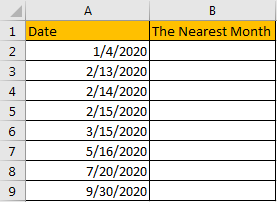## Method: Round Off Date to the Nearest Month by Formula

Step 1: In B2 enter the formula =MONTH(EOMONTH(A2,(DAY(A2)>15)+0)).

In this formula, EOMONTH function returns the date of the last day of a month before or after the specified date; MONTH function returns an integer between 1 and 12 which represents the month in a year. Use the combination of above two functions can round off date to its nearest month.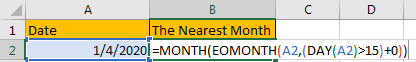Step 2: Press Enter to get returned value. Verify that for date 1/4/2020, the nearest month is January.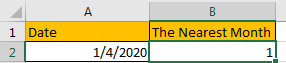Step 3: Drag down the fill handle till reaching the end of the list. Verify that all the nearest months are calculated properly.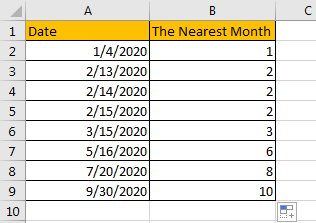Comment:

1. This formula only returns an approximate value, no matter how many days a month, the nearest month for date >15 is the next month.
2. For special month like February, you can adjust the formula to match your request, for example if you want to get 3 (March) for date>13, you can update your formula =MONTH(EOMONTH(A4,(DAY(A4)>13)+0)).

### Related Functions

• Excel MONTH function
The Excel MONTH function returns the month of a date represented by a serial number. And the month is an integer number from 1 to 12. The syntax of the MONTH function is as below:=MONTH (serial_number)…
• Excel DAY function
The Excel DAY function returns a day of a date (from 1 to 31).The DAY function is a build-in function in Microsoft Excel and it is categorized as a DATE and TIME Function.The syntax of the DAY function is as below:= DAY (date_value)…

## How to Convert Date & Time Format to Date in Excel

Sometimes we want to convert date and time format to date only format in excel for example convert `01/29/2019 06:51:03` to `01/29/2019`, we can convert format by Formula or Format Settings. The two ways are easy to learn, so you can select one you like to convert date and time format.

## Convert Date & Time to Date by Formula

Prepare a table with date and time, the format is `MM/DD/YYYY HH/MM/SS`.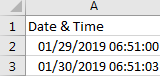If we want to convert them to date format, we can follow below steps:

Step 1: In B2, enter the formula =MONTH(A2) & “/” & DAY(A2) & “/” & YEAR(A2).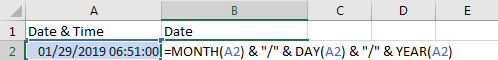Step 2: Click Enter to get the result. Verify that format is changed to date properly, time part is cleared.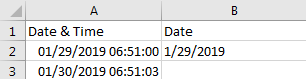Step 3: Drag the Fill Handle down to fill B3. Verify that B3 is changed to date format properly.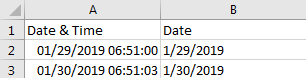## Convert Date & Time to Date by Format Settings

Step 1: On A2, right click to load menu, select Format Cells.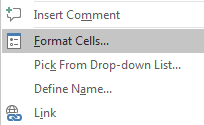Step 2: In Format Cells window, under Number tab, in Category list, select Date; then in Type list select one format you want to show the date. Then the date format is displayed in Sample field.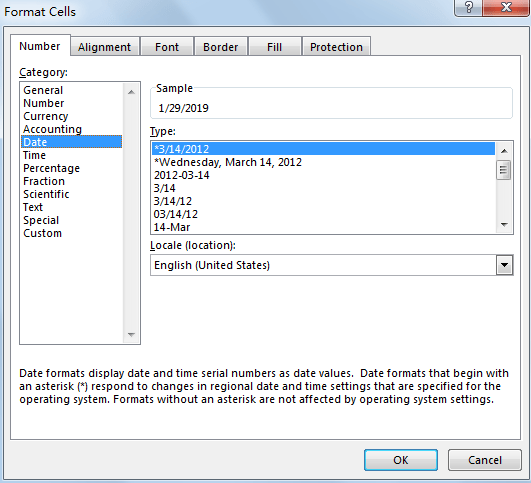Step 3: Click OK. Verify that previous date and time is changed to date format.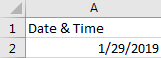### Related Functions

• Excel YEAR function
The Excel YEAR function returns a four-digit year from a given date value, the year is returned as an integer ranging from 1900 to 9999. The syntax of the YEAR function is as below:=YEAR (serial_number)…
• Excel MONTH function
The Excel MONTH function returns the month of a date represented by a serial number. And the month is an integer number from 1 to 12. The syntax of the MONTH function is as below:=MONTH (serial_number)…
• Excel DAY function
The Excel DAY function returns a day of a date (from 1 to 31).The DAY function is a build-in function in Microsoft Excel and it is categorized as a DATE and TIME Function.The syntax of the DAY function is as below:= DAY (date_value)…

## How to Delete or Remove Year from a Date in Excel

This post will guide you how to delete or remove year from a given standard date in Excel. How do I remove the year from a date with a formula in Excel.

## Remove Year from a Date

Assuming that you have a list of data in range B1:B5, in which contain date values. And you want only display the month and Day parts. So you need to remove year from cells. How to do it. You can use a formula based on the MONTH function and the DAY function to achieve the result.  Like this:

`=MONTH(B1)&"-"&DAY(B1)`

Or

`=CONCATENATE(MONTH(B1),"-",DAY(B1))`

Type one of the above formula into cell C1, and press Enter key. And then drag the AutoFill handle from Cell C1 to C5.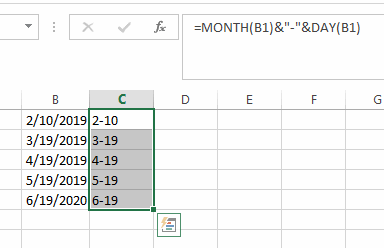You should notice that the year part has been removed for each date in range B1:B5.

You can also use the TEXT function to create a formula to achieve the same result. Like this:

`=TEXT(B1,"mm-dd")`

Type one of the above formula into cell D1, and press Enter key. And then drag the AutoFill handle from Cell D1 to D5.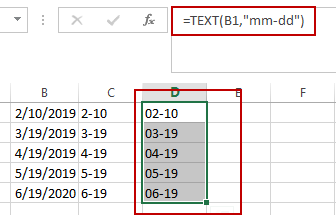### Related Functions

• Excel MONTH function
The Excel MONTH function returns the month of a date represented by a serial number. And the month is an integer number from 1 to 12. The syntax of the MONTH function is as below:=MONTH (serial_number)…
• Excel DAY function
The Excel DAY function returns a day of a date (from 1 to 31).The DAY function is a build-in function in Microsoft Excel and it is categorized as a DATE and TIME Function.The syntax of the DAY function is as below:= DAY (date_value)…
• Excel Text function
The Excel TEXT function converts a numeric value into text string with a specified format. The TEXT function is a build-in function in Microsoft Excel and it is categorized as a Text Function. The syntax of the TEXT function is as below: = TEXT (value, Format code)…

## How to sort Dates by Month and Day Only in Excel

This post will guide you how to sort dates or birthdays by month or day only in Excel. How do I sort dates by Year or Month or Day only with a formula in Excel 2013/2016. How to use the MONTH function and DAY function to sort dates by month or day only in Excel.

## Sort Dates by Month

Assuming that you have a list of data in range A1:B5, which contain date values, and you need to sort those dates by month only. How to achieve it. You can use the MONTH function in combination with a helper column to achieve the result. Just do the following steps:

#1 type the following formula in the adjacent cell of Cell B1, then press Enter key to apply this formula.

`=MONTH(B1)`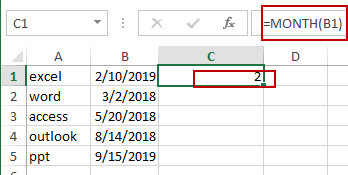#2 drag the AutoFill Handle over to other cells to apply this formula to get the month value from dates.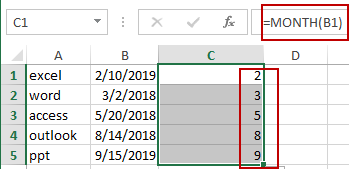#3 select all Month values in helper column. And go to DATA tab, click Sort Smallest to Largest or Sort Largest to Smallest button under Sort & Filter group. The Sort Warning dialog will open.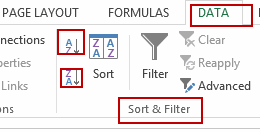#4 select Enable the selection radio button, and click Sort button. You will see that the dates have been sorted by month only. And you can now delete the helper column.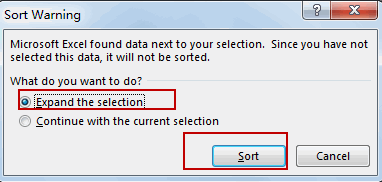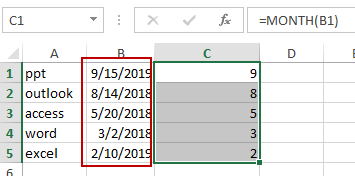## Sort Dates by Year

If you want to sort dates by Year only, you can repeat the above steps, just only use the following formula to instead of Month formula.

`=YEAR(B1)`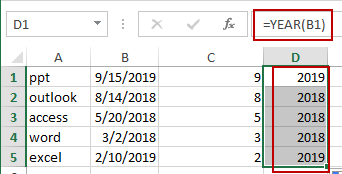## Sort Dates by Day

If you want to sort dates by Day only, you can also repeat the above steps, and just only use the following formula instead of the MONTH formula.

`=DAY(B1)`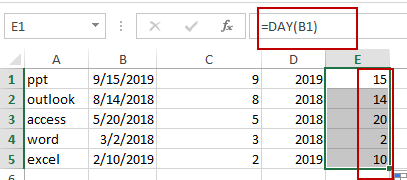Video: Sort Dates by Year/Month/Day

### Related Functions

• Excel YEAR function
The Excel YEAR function returns a four-digit year from a given date value, the year is returned as an integer ranging from 1900 to 9999. The syntax of the YEAR function is as below:=YEAR (serial_number)…
• Excel MONTH function
The Excel MONTH function returns the month of a date represented by a serial number. And the month is an integer number from 1 to 12. The syntax of the MONTH function is as below:=MONTH (serial_number)…
• Excel DAY function
The Excel DAY function returns a day of a date (from 1 to 31).The DAY function is a build-in function in Microsoft Excel and it is categorized as a DATE and TIME Function.The syntax of the DAY function is as below:= DAY (date_value)…

## Excel MONTH Function

This post will guide you how to use Excel MONTH function with syntax and examples in Microsoft excel.

### Description

The Excel MONTH function returns the month of a date represented by a serial number. And the month is an integer number from 1 to 12. And you can use the MONTH function to get a month component from a given date value. And you can feed the month number to another formula.

The MONTH function is a build-in function in Microsoft Excel and it is categorized as a DATE and TIME Function.

The MONTH function is available in Excel 2016, Excel 2013, Excel 2010, Excel 2007, Excel 2003, Excel XP, Excel 2000, Excel 2011 for Mac.

### Syntax

The syntax of the MONTH function is as below:

`=MONTH (serial_number)`

Where the MONTH function arguments is:

• Serial_number -This is a required argument. A valid date value that contains the month component you want to extract. Dates should be entered by using the DATE function, or as results of other formulas or functions. For example, use DATE (2019,3,15) for the 15th day of March, 2019.

Note:

• A serial date is how the Microsoft Excel stores dates and it represents the number of days since 1900-01-01, so the January 1, 1900 date is serial number 1 by default.
• If the serial_number is not a valid Excel date, it will return #VALUE! Error.

### Excel MONTH Function Examples

The below examples will show you how to use Excel MONTH Function to return an integer number that representing the month component of a given Excel date

1# get month of the date in Cell A1, enter the following formula in Cell B1.

`=MONTH(A1)`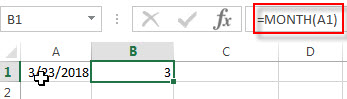2# convert Month name to number, type the following formula in Cell B1.

You should use the DATEVALUE function to convert a date stored as text to a serial number. Then feed the result into the MONTH function to extract a month number from date.

`=MONTH(DATEVALUE(A1 & "1"))`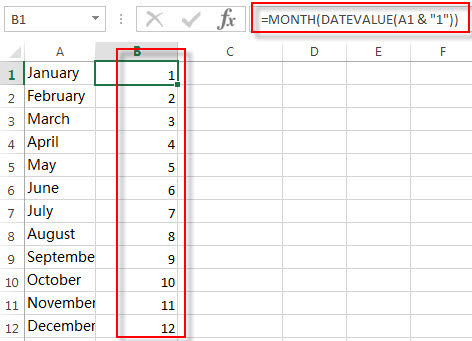### Related Functions

• Excel DAY function
The Excel DAY function returns a day of a date (from 1 to 31). The DAY function is a build-in function in Microsoft Excel and it is categorized as a DATE and TIME Function.The syntax of the DAY function is as below:= DAY (date_value)
• Excel YEAR function
The Excel YEAR function returns a four-digit year from a given date value, the year is returned as an integer ranging from 1900 to 9999. The syntax of the YEAR function is as below:=YEAR (serial_number)…
• Excel DATEVALUE Function
The Excel DATEVALUE function returns the serial number of date. And it can be used to convert a date represented as text format into a serial number that recognizes as a date format.The syntax of the DATEVALUE function is as below:=DATEVALUE(date_text)…

### More Excel Month Function Examples

• Convert Month Name to Number
If you want to convert month name to number with an excel formula, or you want to convert 3 letter month name to numbers, you can create a formual based on the MONTH function and the DATEVALUE function…
• Count Dates in Given Year/Month/Day in Excel
You can create a formula based on the SUMPRODUCT function and the YEAR function to count dates by a give year….

## Excel TODAY Function

This post will guide you how to use Excel TODAY function with syntax and examples in Microsoft excel.

### Description

The Excel TODAY function returns the serial number of the current date. So you can get the current system date from the TODAY function. The serial number is the date-time code used by Excel for date and time calculations.

You can use the TODAY function to get the current date displayed on your worksheet, and regardless of when you open the workbook. And it also can be used for calculating intervals. For example, if you want to know that person’s age (he was born in 1984), you can use the following formula:

=YEAR(TODAY())-1984

This formula will use the TODAY function as an argument for the YEAR function to get the current year, and then subtracts 1984, getting his age.

The TODAY function is a build-in function in Microsoft Excel and it is categorized as a DATE and TIME Function.

The TODAY function is available in Excel 2016, Excel 2013, Excel 2010, Excel 2007, Excel 2003, Excel XP, Excel 2000, Excel 2011 for Mac.

### Syntax

The syntax of the TODAY function is as below:

`=TODAY()`

There are no any arguments for the TODAY function, but you still need to use empty parentheses ().

### Excel TODAY Function Examples

The below examples will show you how to use Excel TODAY Function to return the current system date.

1# using the TODAY function to get the current date, enter the following formula in Cell B1.

`=TODAY()`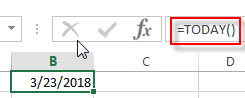2# Get the current date and then subtracts 10 days, using the following formula:

`=TODAY() -10`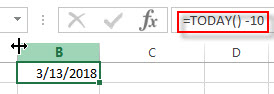3# Get the number of days between the current date and 03/14/2019, type the following formula:

`=DATEVALUE("03/14/2019")-TODAY()`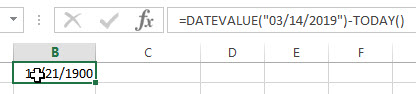4# Get the current day of the month, using the following formula:

`=DAY(TODAY())`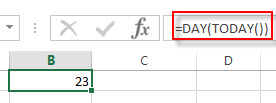5# Get the current month of the year, type the following formula in Cell B1:

`=MONTH(TODAY())`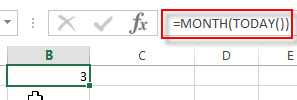You can press F9 key to force the worksheet to recalculate each time and then update the value. If the TODAY function does not update the date when you expect it to, you might need to change the settings that control when the workbook or worksheet recalculates. On the File tab, click Options, and then in the Formulas category under Calculation options, make sure that Automatic is selected.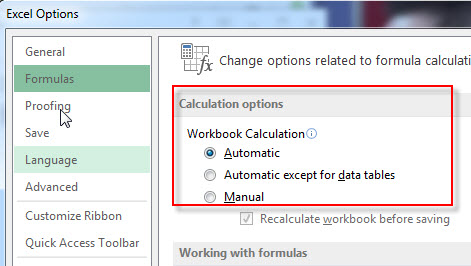### Related Functions

• Excel DAY function
The Excel DAY function returns a day of a date (from 1 to 31). The DAY function is a build-in function in Microsoft Excel and it is categorized as a DATE and TIME Function.The syntax of the DAY function is as below:= DAY (date_value)
• Excel YEAR function
The Excel YEAR function returns a four-digit year from a given date value, the year is returned as an integer ranging from 1900 to 9999. The syntax of the YEAR function is as below:=YEAR (serial_number)…
• Excel MONTH function
The Excel MONTH function returns the month of a date represented by a serial number. And the month is an integer number from 1 to 12. The syntax of the MONTH function is as below:=MONTH (serial_number)…
• Excel DATEVALUE Function
The Excel DATEVALUE function returns the serial number of date. And it can be used to convert a date represented as text format into a serial number that recognizes as a date format.The syntax of the DATEVALUE function is as below:=DATEVALUE(date_text)…

### More Excel TODAY Function Examples

• Conditional Formatting date with red Amber or Green
How to highlight the date with red if the cell dates is past now(). How to highlight the date with amber if the cell date is past now but within the next 6 months from now(). How to highlight the date with green if the cell date is more than 6 months from now…
• Highlight Rows If Dates Have Passed
Assuming that you have a worksheet that have a bunch of columns and each row has a date column. If the date in the date column goes past from now on and you want to highlight the entire row with red color….
• Count Dates in Given Year/Month/Day in Excel
You can create a formula based on the SUMPRODUCT function and the YEAR function to count dates by a give year….

## How to Add / Subtract Days, Months and Years to Date

This post will guide you how to add or subtract a given number of days to a date in excel. How to add or subtract weeks to a date using excel formula. How to add or subtract a certain number of months to a date using excel formula. How to add or subtracts a given number of years to a date using an excel formula.  And how to add or subtract a combination of years, months and days to a date.

Assuming that you have a list of date in your worksheets, and you want to perform some arithmetic operations (such as, addition or subtraction) to calculate different time units, such as, you want to add or subtract a number of days to or from a date by using a formula to adjust the date according to your needs.

## Add / subtract days to date

If you have a list of date in a range or worksheet, and you want to add or subtract a certain number of days to the date in the range, you can refer to the following general formula:

`=Date + days`

Or

`=Date - days`

For example, to add 100 days to a date in Cell B1, then you can write the following formula in Cell C1:

=B1 +100

To subtract 100 days from a date in Cell B1, then you can write the following formula in Cell D1

=B1-100

If you want to add or subtract 100 days to a date in Cell b1, then you can use the TODAY function to build the following formula:

=Today() +100

Or

=Today() – 100

Let’s see the below screenshot to see the returned result by the above formulas: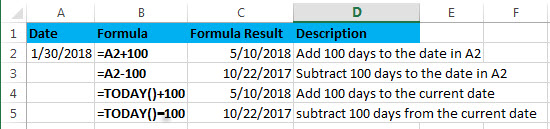## Add / subtract weeks to date

Assuming that you want to add or subtract a given number of weeks to a certain date in A2, then you can refer to the following generic formula:

`=Date +N Weeks * 7  `

Or

`=Date – N weeks*7`

You need to multiply the number of weeks by 7 to get the total number of days, then you can perform arithmetic operations in the same time unit (days).

Let’s see the below examples:

Supposing that you want to add or subtract 5 weeks to a date in A2, you can refer to the following excel formulas:

=A2 + 5*7

=A2 – 5*7

=TODAY() + 5*7

=TODAY() – 5*7

Let’s see the returned results by the above formula from the below screenshot: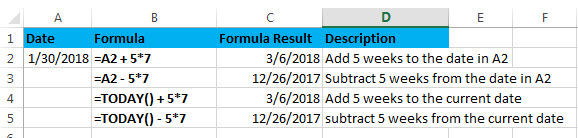## Add / subtract months to date

If you want to add or subtract a given number of whole months to a date, you can use the EDATE function to create a generic formula as follows:

`=EDATE(start_date, months)`

Start_date – the start date

Months – the number of months that you want to add or subtract

If you want to subtract months, you just need to enter a negative number as the months argument in the EDATE function.

Assuming that you want to add or subtract 3 months to the date in A2, you can use the following formula:

=EDATE(A2,3)

=EDATE(A2,-3)

=EDATE(TODAY(),3)

=EDATE(TODAY(),-3)

Let’s see the last result for above formulas: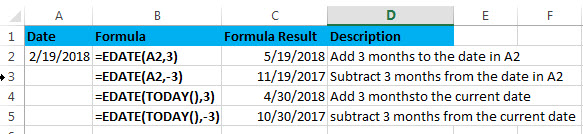## Add / subtract years to date

If you want to add or subtract a given number of years to a date, you can create an excel formula based on the DATE function, the YEAR function, the MONTH function and the DAY function.

The generic formula is as follows:

To add years to a date in excel:

`=DATE(YEAR(date)+ years, MONTH(date),DAY(date))`

To subtract years to a date in excel:

`=DATE(YEAR(date)+ years, MONTH(date),DAY(date))`

Assuming that you want to add or subtract 2 years to a date in A2, you can use the following formulas:

=DATE(YEAR(A2)+ 2, MONTH(A2),DAY(A2))

or

=DATE(YEAR(A2)- 2, MONTH(A2),DAY(A2))

Let’s see how this formula works:

=YEAR(A2)

Result: 2018

The YEAR function returns the year component of a date in A2.

=MONTH(A2)

Result: 2

The MONTH function returns the month portion of a date in A2.

=DAY(A2)

Result: 19

The DAY function returns the day of component of a date in A2.

Let’s see the following screenshot to show the result for above DATE formulas: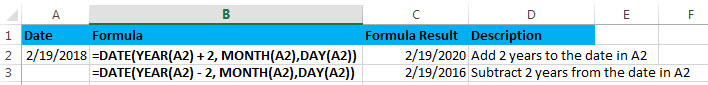## Add / subtract a combination of days, months and years

If you want to add or subtract a combination of days, months and years to a date in a single formula, you can also use a combination of the DATE function, the YEAR function, the MONTH function and the DAY function.

To add days, months and years in excel:

`=DATE(YEAR(start_date) + years, MONTH(start_date) + months, DAY(start_date) + days)`

To subtract days, months and years

`=DATE(YEAR(start_date) - years, MONTH(start_date) - months, DAY(start_date) - days)`

Assuming that you want to add or subtract 2 years, 3 months and 100 days to or from a date in cell A2, you can use the following formulas:

=DATE(YEAR(A2) + 2, MONTH(A2) + 3, DAY(A2) + 100)

Or

=DATE(YEAR(A2) – 2, MONTH(A2) – 3, DAY(A2) – 100)

Then enter the above formula into Cell C2 or C3, then let’s see the returned results: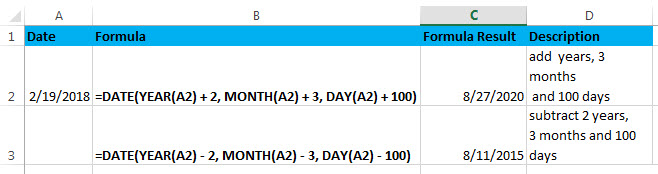### Related Formulas

• Convert date to month and year only in excel
If you want to convert the date to month and year only, you can use “yyyymm” format code within the TEXT function in excel, so you can write down the below TEXT formula:=TEXT(date,”yyyymm”)
• Convert date to month and day only in excel
If you want to convert the date (mm/dd/yyyy) to month and day only, you can use “mm dd” format code within the TEXT function in excel, so you can write down the below TEXT formula: =TEXT(date,”mm dd”)
• Convet Text Date dd/mm/yy to mm/dd/yyyy as Date Format
If you want to convert dates in the text format to the date format as mm/dd/yyyy, you can use a combination of the DATE function, the VALUE function, the RIGHT function, the MID function, and the LEFT function to create a new excel formula…

### Related Functions

• Excel DAY function
The Excel DAY function returns a day of a date (from 1 to 31).The DAY function is a build-in function in Microsoft Excel and it is categorized as a DATE and TIME Function.The syntax of the DAY function is as below:= DAY (date_value)…
• Excel EDATE function
TThe Excel EDATE function returns the serial number that represents the date that is a specified number of months before or after a specified date.The syntax of the EDATE function is as below:=EDATE (start_date, months)…
• Excel DATE function
The Excel DATE function returns the serial number for a date.The syntax of the DATE function is as below:= DATE (year, month, day)…
• Excel TODAY function
The Excel TODAY function returns the serial number of the current date. So you can get the current system date from the TODAY function. The syntax of the TODAY function is as below:=TODAY()…
• Excel MONTH Function
The Excel MONTH function returns the month of a date represented by a serial number. And the month is an integer number from 1 to 12.The syntax of the MONTH function is as below:=MONTH (serial_number)…

## Excel Day Function

This post will guide you how to use Excel DAY function with syntax and examples in Microsoft excel.

### Description

The Excel DAY function returns a day of a date (from 1 to 31)

The DAY function is a build-in function in Microsoft Excel and it is categorized as a DATE and TIME Function.

The DAY function is available in Excel 2016, Excel 2013, Excel 2010, Excel 2007, Excel 2003, Excel XP, Excel 2000, Excel 2011 for Mac.

### Syntax

The syntax of the DAY function is as below:

`= DAY (date_value)`

### Example

The below examples will show you how to use Excel DAY Function to return the day of a date.

#1 =DAY(B2)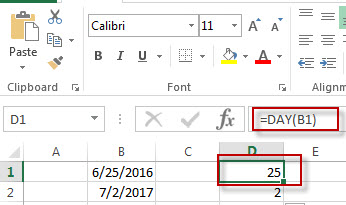Note:  The above excel formula will return the day of the date in Cell B1.

### Related Functions

• Excel YEAR function
The Excel YEAR function returns a four-digit year from a given date value, the year is returned as an integer ranging from 1900 to 9999. The syntax of the YEAR function is as below:=YEAR (serial_number)…
• Excel MONTH function
The Excel MONTH function returns the month of a date represented by a serial number. And the month is an integer number from 1 to 12. The syntax of the MONTH function is as below:=MONTH (serial_number)…
• Excel DATEVALUE Function
The Excel DATEVALUE function returns the serial number of date. And it can be used to convert a date represented as text format into a serial number that recognizes as a date format.The syntax of the DATEVALUE function is as below:=DATEVALUE(date_text)…
• Excel WEEKDAY function
The Excel WEEKDAY function returns a integer value representing the day fo the week for a given Excel date and the value is range from 1 to 7.The syntax of the WEEKDAY function is as below:=WEEKDAY (serial_number,[return_type])…